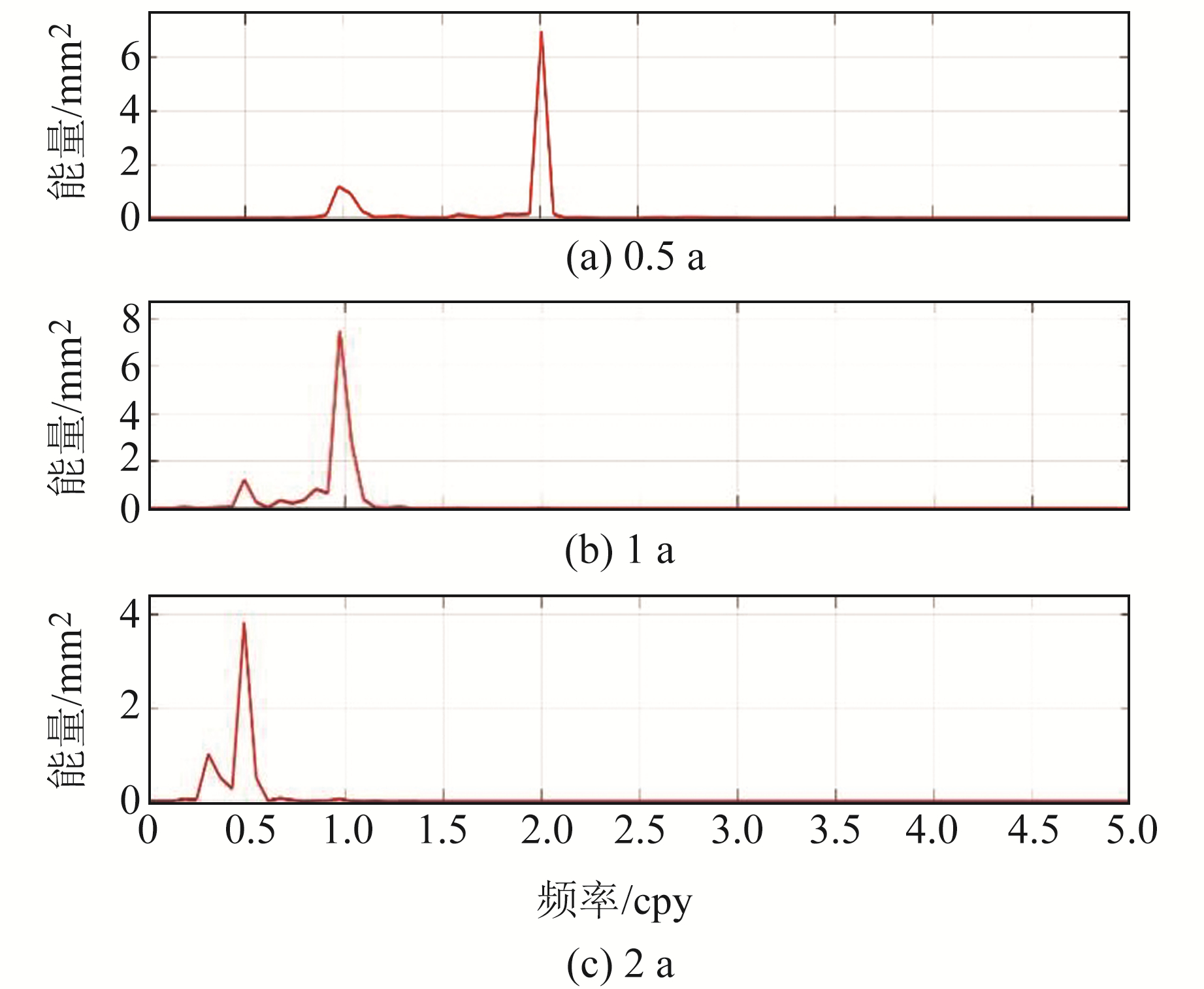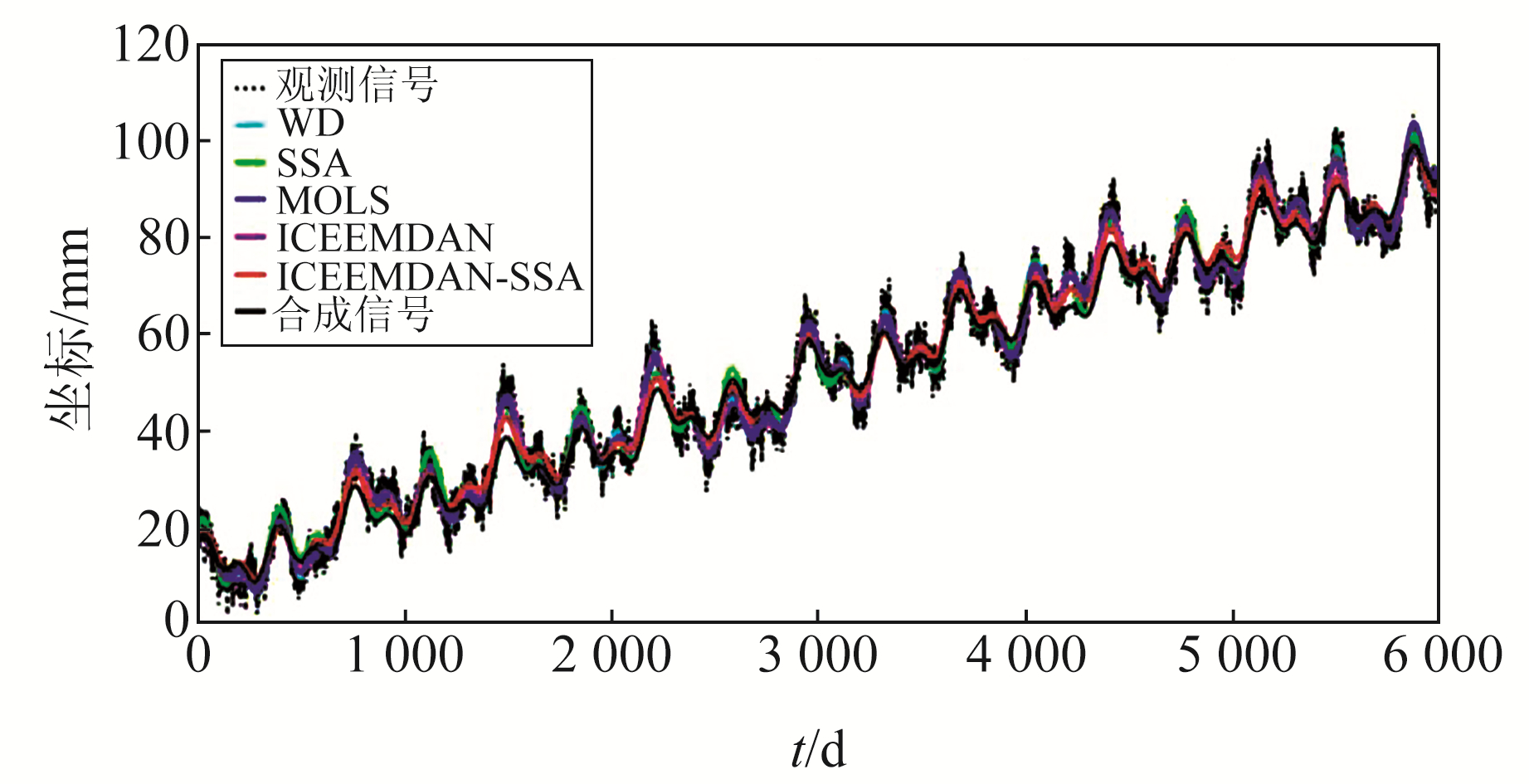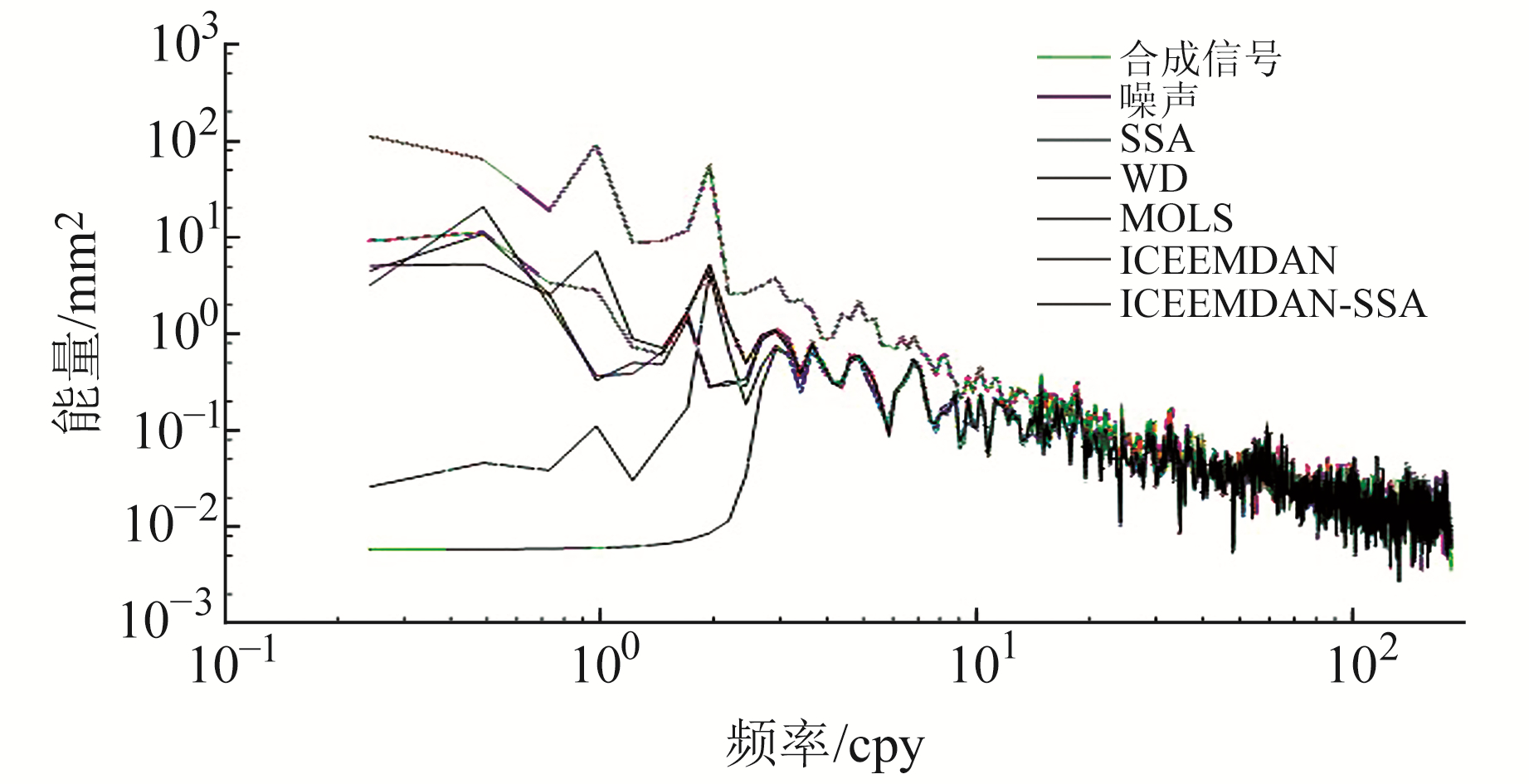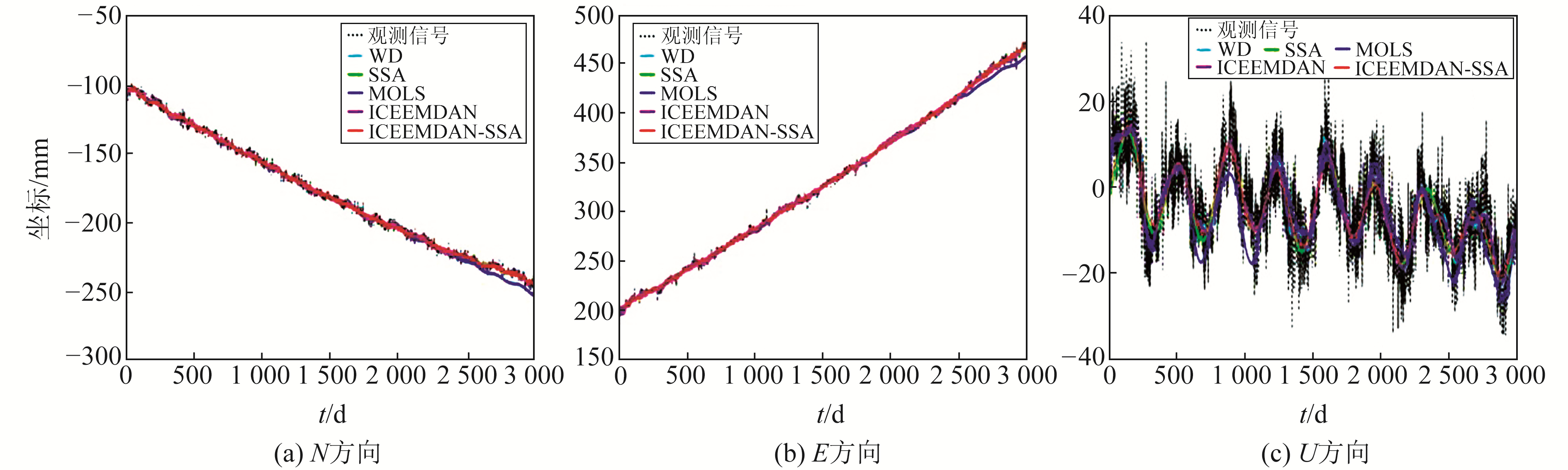﻿ 基于改进的经验模态分解和SSA联合算法的GPS坐标时间序列重构文章快速检索 高级检索
 大地测量与地球动力学2022, Vol. 42Issue (9): 904-909  DOI: 10.14075/j.jgg.2022.09.005### 引用本文ZHANG Yilei, BIAN Jiawen, DING Kaihua, et al. GPS Coordinate Time Series Reconstruction Based on ICEEMDAN and SSA Joint Algorithm[J]. Journal of Geodesy and Geodynamics, 2022, 42(9): 904-909.### Foundation support

National Natural Science Foundation of China, No. 42174012, 62072163.

### Corresponding author

BIAN Jiawen, associate professor, major in signal and information processing, probability statistics and its applications, E-mail: jwbian@cug.edu.cn.

### About the first author

ZHANG Yilei, postgraduate, major in time series analysis and statistical information processing, E-mail: after_24hours@163.com.

### 文章历史

1. 中国地质大学(武汉)数学与物理学院，武汉市鲁磨路388号，430074;
2. 中国地质大学(武汉)地理与信息工程学院，武汉市鲁磨路388号，430074;
3. 湖北经济学院信息管理与统计学院，武汉市杨桥湖大道8号，430205

GPS坐标时间序列主要包括长期趋势项、周期项以及噪声项，对GPS坐标时间序列进行精确重构，不仅可以提取该站点的周期性信号，还可以准确估计噪声振幅、速度不确定度等参数。近年来，国内外学者提出多种方法估计时变振幅的周期信号，如奇异谱分析法(singular spectrum analysis, SSA)、小波分解法(wavelet decomposition, WD)、滑动最小二乘法(moving ordinary least squares, MOLS)以及经验模态分解法(empirical mode decomposition, EMD)等。其中，EMD法本身存在模态混叠，且在提取闪烁噪声环境下的周期信号时存在吸收噪声的现象，会导致重构精度降低。

1 具有时变振幅的GPS坐标时间序列模型

GPS坐标时间序列一般由长期趋势、周期项以及噪声项组成，一般建模如下：

 $y(t)=h_0+v t+s(t)+\varepsilon(t), t=1, 2, \cdots, N$ (1)

 \begin{aligned} s(t)=& \sum\limits_{i=1}^3 a_i(t) \cos \left(2 \pi f_i t\right)+\\ & b_i(t) \sin \left(2 \pi f_i t\right) \end{aligned} (2)

 $a_i(t)=a_i+{\mathrm{e}}^{p \sin (t)}, b_i(t)=b_i+{\mathrm{e}}^{q \sin (t)}$ (3)

2 基于ICEEMDAN-SSA的GPS坐标时间序列分解和重构

1) 输入GPS坐标时间序列x，通过EMD法进行第i次迭代(i=1, 2, …, I)，迭代公式如下：

 $x^{(i)}=x+\beta_0 E_1\left(w^{(i)}\right)$ (4)

 $r_1=\frac{1}{I} \sum\limits_{i=1}^I M\left(x^{(i)}\right)$ (5)

 ${\mathrm{IMF}}_1=x-r_1$ (6)

2) 第二个残差序列r2以及第二个模态IMF2计算公式如下：

 $r_2=\frac{1}{I} \sum\limits_{i=1}^I M\left(r_1+\beta_1 E_2\left(w^{(i)}\right)\right)$ (7)
 ${\mathrm{IMF}}_2=r_1-r_2$ (8)

3) 循环计算IMFkrk，公式如下：

 $r_k=\frac{1}{I} \sum\limits_{i=1}^T M\left(r_{k-1}+\beta_{k-1} E_k\left(w^{(i)}\right)\right)$ (9)
 ${\mathrm{IMF}}_k=r_{k-1}-r_k$ (10)

4) 得到Q个不同时间尺度下的模态{IMF1, IMF2, …, IMFQ}，通过计算各模态分量与原GPS信号的相关系数和方差贡献率筛选出噪声模态和信号模态，此时有：

 $x=\sum\limits_{i=1}^q {\rm{IMF}}_i({\rm { noise }})+\sum\limits_{j=q+1}^Q {\mathrm{IMF}}_j(\rm{signal})$ (11)

5) 将各信号模态分别排成Hankel矩阵Ds(L, K) 形式，其中s为第s个信号模态分量(s=q+1, q+2, …, Q)，L=N-K+1≤N/2：

 ${\boldsymbol{D}}^s=\left[\begin{array}{ccccc} x_1^s & x_2^s & x_3^s & \cdots & x_K^s \\ x_2^s & x_3^s & x_4^s & \cdots & x_{K+1}^s \\ x_3^s & x_4^s & \ddots & \cdots & x_{K+2}^s \\ \vdots & \vdots & \vdots & \ddots & \vdots \\ x_L^s & x_{L+1}^s & x_{L+2}^s & \cdots & x_N^s \end{array}\right]$ (12)

 ${\boldsymbol{C}}_j=\frac{1}{N} {\boldsymbol{D}}_j^{\mathrm{T}} {\boldsymbol{D}}_j$ (13)

Cj进行特征值分解得到各特征值λij和特征向量Eij，将特征值降序排列后计算得到各主成分aij，表达式如下：

 $a_i^j=\sum\limits_{m=1}^M x_{i+m} E_m^j, 0 \leqslant i \leqslant N-L$ (14)

6) 计算重建成分：

 $\begin{array}{l} x_i^p=\\ \left\{\begin{array}{l} \frac{1}{i} \sum\limits_{j=1}^i a_{i-j}^p E_j^p, 1 \leqslant i \leqslant L-1 \\ \frac{1}{L} \sum\limits_{j=1}^L a_{i-j}^p E_j^p, L \leqslant i \leqslant N-L+1 \\ \frac{1}{N-i+1} \sum\limits_{j=i-N+L}^L a_{i-j}^p E_j^p, N-L+2 \leqslant i \leqslant N \end{array}\right. \end{array}$ (15)

7) 根据特征值贡献率选取前p个重建成分累加得到重建后的模态{IMFq+1R, …, IMFQR}，并计算得到重构信号xD

 $x^D={\mathrm{IMF}}_{q+1}^R+\cdots+{\mathrm{IMF}}_Q^R$ (16)
3 模拟实验分析 3.1 模拟实验设置

3.2 实验结果分析图 1 ICEEMDAN方法提取的周期信号 Fig. 1 Periodic signals extracted by the ICEEMDAN method图 2 基于ICEEMDAN和ICEEMDAN-SSA提取周期信息与趋势信息 Fig. 2 Periodic information and trend information extracted by ICEEMDAN and ICEEMDAN-SSA图 3 闪烁噪声振幅为10 mm/${{\rm{a}}^{ - \frac{k}{4}}}$时各个估计方法得到的GPS信号 Fig. 3 GPS signal obtained by various estimation methods with noise amplitude of 10 mm/${{\rm{a}}^{ - \frac{k}{4}}}$图 4 闪烁噪声振幅为10 mm/${{\rm{a}}^{ - \frac{k}{4}}}$时各个方法得到的PSD Fig. 4 PSD obtained by various estimation methods with noise amplitude of 10 mm/${{\rm{a}}^{ - \frac{k}{4}}}$表 1 闪烁噪声振幅为10 mm/${{\rm{a}}^{ - \frac{k}{4}}}$时各方法的指标估计值 Tab. 1 Index values of various estimation methods with noise amplitude of 10 mm/${{\rm{a}}^{ - \frac{k}{4}}}$

4 实际数据分析表 2 GPS站点信息 Tab. 2 GPS station information图 5 KUNM站各方向时间序列重构结果 Fig. 5 Reconstruction results of time series in each direction of KUNM station表 3 通过不同方法得到KUNM站点各指标值 Tab. 3 Index values of KUNM station with different methods表 4 ICEEMDAN-SSA在KUNM站NEU方向的重建结果与SSA、WD、MOLS、ICEEMDAN重建结果之间的相关系数 Tab. 4 Correlation coefficients between the reconstruction result of ICEEMDAN-SSA and SSA, WD and MOLS in the N, E and U direction of KUNM station
5 结论

  Chen Q, Dam T, Sneeuw N, et al. Singular Spectrum Analysis for Modeling Seasonal Signals from GPS Time Series[J]. Journal of Geodynamics, 2013, 72: 25-35 DOI:10.1016/j.jog.2013.05.005 (0)  Bogusz J. Geodetic Aspects of GPS Permanent Station Non-Linearity Studies[J]. Acta Geodynamica et Geomaterialia, 2015, 12(4): 323-333 (0)  Klos A, Bos M S, Bogusz J. Detecting Time-Varying Seasonal Signal in GPS Position Time Series with Different Noise Levels[J]. GPS Solutions, 2018, 22(1) (0)  张双成, 李振宇, 何月帆, 等. GNSS高程时间序列周期项的经验模态分解提取[J]. 测绘科学, 2018, 43(8): 80-84 (Zhang Shuangcheng, Li Zhenyu, He Yuefan, et al. Extracting of Periodic Component of GNSS Vertical Time Series Using EMD[J]. Science of Surveying and Mapping, 2018, 43(8): 80-84) (0)  Torres M E, Colominas M A, Schlotthauer G, et al. A Complete Ensemble Empirical Mode Decomposition with Adaptive Noise[C]. 2011 IEEE International Conference on Acoustics, Speech and Signal Processing, Prague, 2011 (0)  Colominas M A, Schlotthauer G, Torres M E. Improved Complete Ensemble EMD: A Suitable Tool for Biomedical Signal Processing[J]. Biomedical Signal Processing and Control, 2014, 14(1): 19-29 (0)  Pan Y J, Shen W B, Ding H, et al. The Quasi-Biennial Vertical Oscillations at Global GPS Stations: Identification by Ensemble Empirical Mode Decomposition[J]. Sensors, 2015, 15(10): 26 096-26 114 DOI:10.3390/s151026096 (0)  Scaife A A, Athanassiadou M, Andrews M, et al. Predictability of the Quasi-Biennial Oscillation and Its Northern Winter Teleconnection on Seasonal to Decadal Timescales[J]. Geophysical Research Letters, 2014, 41(5): 1 752-1 758 (0)  Bos M S, Fernandes R M S, Williams S D P, et al. Fast Error Analysis of Continuous GPS Observations[J]. Journal of Geodesy, 2008, 82(3): 157-166 DOI:10.1007/s00190-007-0165-x (0)  Gazeaux J, Williams S, King M, et al. Detecting Offsets in GPS Time Series: First Results from the Detection of Offsets in GPS Experiment[J]. Journal of Geophysical Research: Solid Earth, 2013, 118(5): 2 397-2 407 (0)  吴浩, 卢楠, 邹进贵, 等. GNSS变形监测时间序列的改进型3σ粗差探测方法[J]. 武汉大学学报: 信息科学版, 2019, 44(9): 1 282-1 288 (Wu Hao, Lu Nan, Zou Jingui, et al. An Improved 3σ Gross Error Detection Method for GNSS Deformation Monitoring Time Series[J]. Geomatics and Information Science of Wuhan University, 2019, 44(9): 1 282-1 288) (0)  Liu N, Dai W J, Santerre R, et al. A MATLAB-Based Kriged Kalman Filter Software for Interpolating Missing Data in GNSS Coordinate Time Series[J]. GPS Solutions, 2017, 22(1) (0)
GPS Coordinate Time Series Reconstruction Based on ICEEMDAN and SSA Joint Algorithm
ZHANG Yilei1BIAN Jiawen1DING Kaihua2     RAN Jianuo1     LIU Wenping3
1. School of Mathematics and Physics, China University of Geosciences, 388 Lumo Road, Wuhan 430074, China;
2. School of Geography and Information Engineering, China University of Geosciences, 388 Lumo Road, Wuhan 430074, China;
3. School of Information Management and Statistics, Hubei University of Economics, 8 Yangqiaohu Avenue, Wuhan 430205, China
Abstract: Based on the ability of ICEEMDAN algorithm to accurately separate and extract low-frequency signals and trend information without a priori information, and the advantage of SSA with better signal reconstruction, we propose a joint reconstruction method based on ICEEMDAN and SSA. The method extracts and reconstructs the weak periodic signals by using ICEEMDAN method; this makes up for a deficiency of the SSA method, namely that it is difficult to extract periodic signals when the singular values of the Hankel matrix corresponding to the weak periodic signals are close to the singular values of the noisy Hankel matrix, which are easily masked by noise. We verify the decomposition and reconstruction accuracy of the algorithm by simulated experiments and real site data. We further compare the algorithm with the singular spectrum analysis, wavelet decomposition, and moving ordinary least squares methods. The experimental results show that the joint ICEEMDAN-SSA algorithm has better reconstruction accuracy compared with existing methods.
Key words: GPS coordinate time series; periodic signal; ICEEMDAN algorithm; signal reconstruction